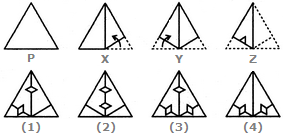# Non Verbal Reasoning - Paper Cutting - Discussion

### Discussion :: Paper Cutting - Section 1 (Q.No.9)

Each of the following questions consists of a set of three figures X, Y and Z showing a sequence of folding of apiece of paper. Figure (Z) shows the manner in which the folded paper has been cut. These three figures are followed by four answer figures from which you have to choose a figure which would most closely resemble the unfolded form of figure (Z).

9.

Choose a figure which would most closely resemble the unfolded form of Figure (Z).[A]. 1 [B]. 2 [C]. 3 [D]. 4

Explanation:

No answer description available for this question.

 M11 said: (Feb 14, 2014) Direction of curves in not clearly given however using elimination of options you can got the answer.

 Bidur said: (Aug 19, 2016) One step of direction is not given.